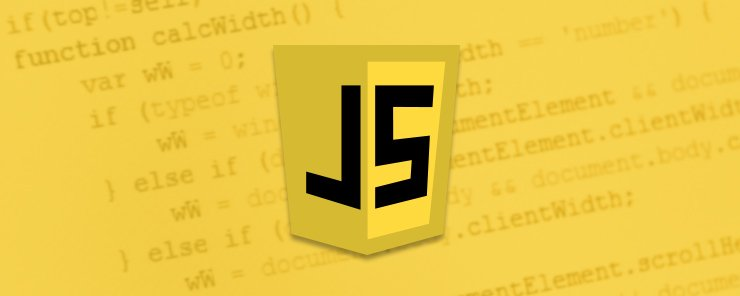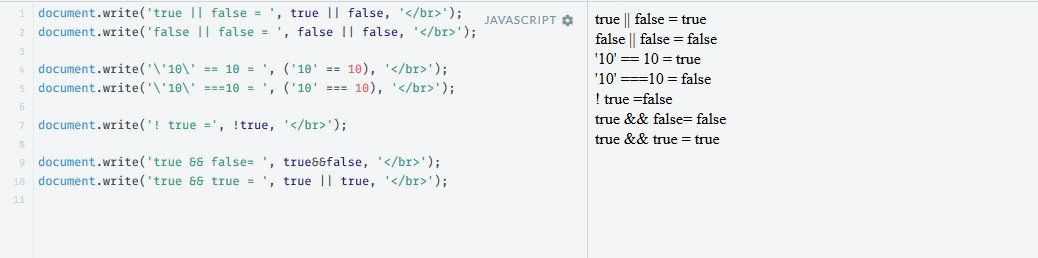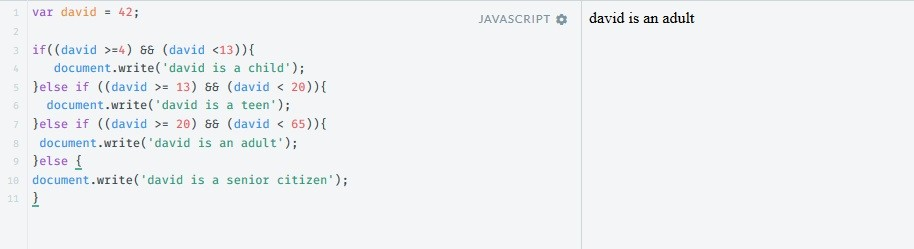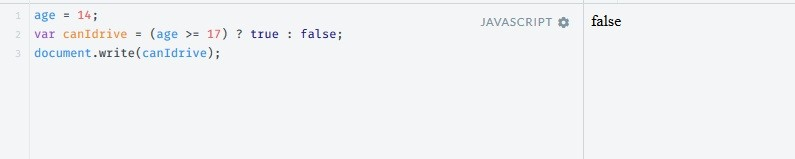Web Programming TutorialsLearn about Logical Operators in JavaScript

# Learn about Logical Operators in JavaScriptLogical operators include ‘&&'(AND), ‘||’ (OR) and ‘!'(is NOT). They are used in conditional statements – statements which give the green light for actions to be performed only under certain circumstances. The action/s will be performed only if the statement equals to the Boolean

value of TRUE.
|| – the condition/s must be TRUE on at least one side of this operator in order for the whole statement to yield TRUE.
&& – the conditions on both sides of this operator must be TRUE in order for the whole statement to yield TRUE.
! – Yields true if the value attached to it is equal to FALSE.

Let’s learn how they work by demonstrating their functionality:

The OR operator:

```document.write('true || false =', true || false, '</br>');
document.write('false || false =', false || false, '</br>');
```

The EQUALS operator:

```document.write('\'10\' == 10 = ', ('10' == 10), '</br>');
document.write('\'10\' ===10 = ', ('10' === 10), '</br>');
```

The is NOT operator:

`document.write('! true =', !true, '</br>');`

The AND opertor:

```document.write('true && false=', true&&false, '</br>');
document.write('true && true =', true || true, '</br>');
```A working example of the && operator:

```var david = 42;
if((david >=4) && (david <13)){
document.write('david is a child');
}else if ((david >= 13) && (david < 20)){
document.write('david is a teen');
}else if ((david >= 20) && (david < 65)){
document.write('david is an adult');
}else {
document.write('david is a senior citizen');
}
```

outcome:Explanation: the code first checks if David is under or equal to 4 AND smaller than 13. if both conditions are met, it carries out the actions inside the following ‘{}’. otherwise (if the outcome is FALSE, which it is in our case), it goes to the next conditional statement, and checks if David is bigger than or equal to 13 AND smaller than 20. if both conditions are met, it carries out the actions inside the following ‘{}’. otherwise (FALSE outcome, like in this specific case), it goes to the next conditional statement, and checks if david is bigger than or equal to 20 AND smaller than 65. In our case, 42 is bigger than 20 AND smaller than 65 (both sides of the && yield TRUE), so the actions inside the following ‘{}’ are carried out.

Working example of OR:

```var kitty = '#fff';
if((kitty == 'white') || (kitty == '#fff')){
}else {
document.write('kitty can hear');
}
```

Another way to perform logical conditions is with the ternary operator, like so:
(condition) ? Do if TRUE : Do if FALSE;

The ternary operator starts with a condition followed by a question mark. If the condition is TRUE, the actions immediately following the question mark will be performed (or the value will be assigned). Otherwise, the actions on the other (right) side of the “:” will be performed (or the value will be assigned).A working example:

```age = 14;
var canIdrive = (age >= 17) ? true : false;
```

In this case, the variable canIdrive will get the value of TRUE if it’s bigger than or equal to 17, otherwise it will get the value of FALSE.To summarize, we’ve seen Javascript logical operators in action. We use the logical operators to check if multiple conditions are TRUE or FALSE (i.e., check the value of more than one variable or check if several conditions apply to the same variable) . They are are extremely useful inside if/else as well as while statements, and simple to use. That’s all for today, See you in next tutorial on JavaScript till then keep learning

### Build A Front End Of an e-Commerce Site Using React

21,501Fans
4,213Followers
104,000Subscribers

### Build A Front End Of an e-Commerce Site Using React

- Advertisement -